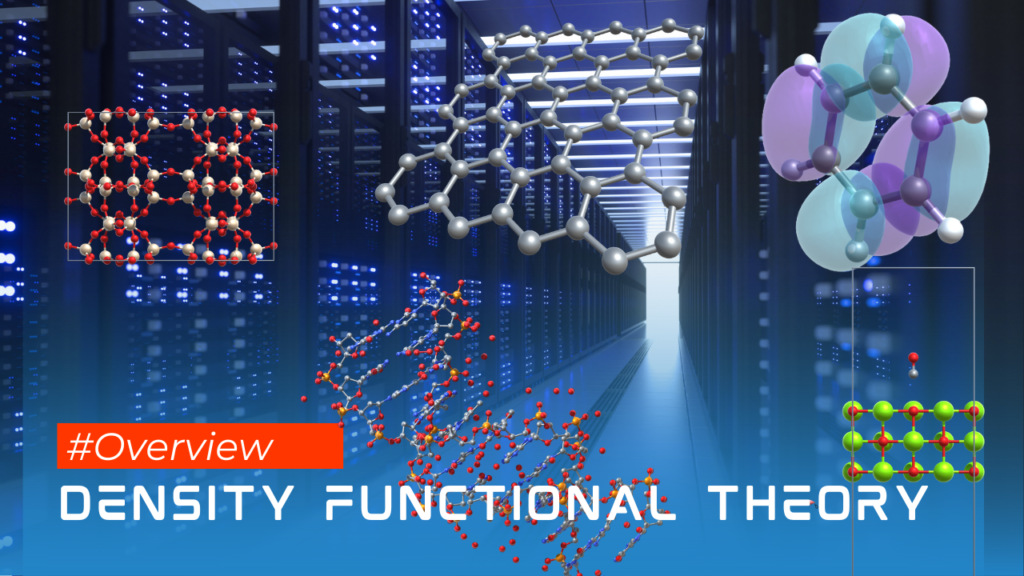# A quick introduction to Density Functional TheoryDensity functional theory (DFT) is a computational quantum mechanical modeling method used in physics, chemistry and materials science to investigate the electronic structure (or nuclear structure) (principally the ground state) of many-body systems, in particular atoms, molecules, and the condensed phases.

DFT is a universal approach that can be applied to many different systems, including both periodic and non-periodic systems. It is a formally exact theory, meaning that it is based on well-defined mathematical principles and that it can, in principle, provide an exact description of the electronic structure of a many-body system. In practice, DFT is implemented using approximate functionals, which introduce some errors into the calculations, but these errors can often be well controlled.

DFT has become a widely used method for studying the electronic structure of many-body systems, due to its computational efficiency and accuracy. It has been applied to a wide range of systems, including atoms, molecules, solids, and extended systems, such as surfaces and interfaces.

One of the key equations in DFT is the Kohn-Sham equation, which is a single-particle Schrödinger equation that is used to describe the behavior of the electrons in a many-body system. The Kohn-Sham equations take the form:$\left[-\frac{\hbar^2}{2m}\nabla^2 + v_{\text{ext}}(\mathbf{r}) + v_{\text{H}}(\mathbf{r}) + v_{\text{xc}}(\mathbf{r})\right] \psi_i(\mathbf{r}) = \epsilon_i \psi_i(\mathbf{r})$

where$v_{\text{ext}}(\mathbf{r})$ is the external potential (such as the potential from the nuclei),$v_{\text{H}}(\mathbf{r})$ is the Hartree potential (which describes the electrostatic interaction between the electrons), and$v_{\text{xc}}(\mathbf{r})$ is the exchange-correlation potential (which describes the many-body effects in the system). The wavefunction$\psi_i(\mathbf{r})$ and energy$\epsilon_i$ are the solutions to the Kohn-Sham equation for the$i$th electron.

To solve the Kohn-Sham equation, one typically uses a computational software package. There are many different software packages available for performing DFT calculations, including:

• TURBOMOLE
• VASP (Vienna Ab initio Simulation Package)
• Quantum Espresso
• ABINIT
• Gaussian
• NWChem

Each of these software packages has its own strengths and limitations, and choosing the right one for a particular calculation can be a complex task.

When choosing a software package for Density Functional Theory (DFT) calculations, it is important to consider a few key factors. Firstly, it is important to consider the specific needs of your research project, as different software packages may have different strengths and capabilities. For example, some packages may be better suited to certain types of materials (molecular or periodic) or calculations (DFT or TDDFT), so it is important to choose a package that is well-suited to your specific needs. Additionally, it is also important to consider the overall ease of use and user-friendliness of the software, as well as its performance and accuracy. Additionally, it may also be helpful to consider factors such as the availability of user support and documentation, as well as the overall cost of the software.

[wpedon id="7041" align="center"]

This site uses Akismet to reduce spam. Learn how your comment data is processed.